# Graber: Rationally Connected Varieties

Introduction

Definition. A field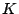is quasi-algebraically closed (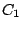) if any polynomial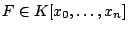with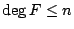has a root in.

Any finite field is quasi-algebraically closed, as is any function field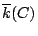of a curve over an algebrically closed field. This implies that any Laurent series ring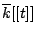is.

These generalize to the following three possible definitions:

Definition. A projective variety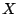is rationally connected (RC) if any two general points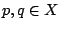can be joined by a rational curve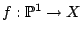.

Definition.is rationally chain connected (RCC) if any two general pointscan be joined by a chain of rational curves.

Definition.is separably rationally connected (SRC) ifis normal and there exists a rational curve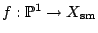such that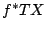is ample, i.e.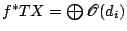,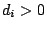.

Theorem. Ifis smooth projective over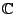, thenis rationally connected (RC) if and only ifis rationally chain connected (RCC) if and only ifis separably rationally connected (SRC).

In characteristic zero,a smooth hypersurface of degree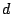in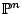is rationally connected if and only if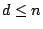.

The main result we will consider is the following:

Theorem. [G, Harris, Starr] If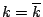,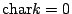, then any rationally connected variety over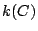for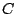a curve has a rational point.

Remark. In characteristic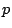, the same is true for SRC. (de Jong, Starr)

Theorem. Over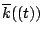, any rationally connected variety has a rational point.

This follows from the function field case. Geometrically, you can find a nonsingular integral model over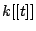; to find a section, it is equivalent to find a reduced component of the central fiber.

Theorem. [Ernault] If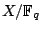is smooth, projective, and geometrically rationally chain connected, thenhas a rational point.

Proof, a Beginning

We now prove the theorem that an RC variety overhas a rational point.

Choose an integral model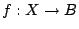. First, we reduce to the case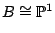. By restriction of scalars, have a map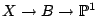; the fibres of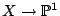are products of fibres of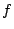, and the product of rationally connected varieties is rationally connected.

Next, choose a curve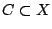which dominates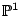. Deformand specialize until it breaks off a section. We have a map of moduli spaces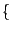curves in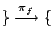coverings of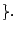The latter is built out the data of the branch points plus monodromy; fixing the genus of the curve and the degree of the cover, we know that this moduli space is irreducible. It is possible to degenerate any branched cover ofto a reducible cover with a section as one component. It is enough to show that this map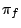on moduli spaces is surjective. Now it is just a matter of tracing the monodromy as the branch points move around (at least in characteristic zero). In characteristic, it can also be done (look, for example, at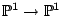).

To make all of this more precise, we look at stable maps. Givena smooth projective variety over, and given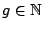and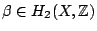, we construct a space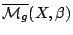consisting of maps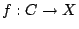such thatis a connected nodal curve of arithmetic genus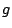,is a morphism, and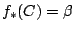, together with a stability criterion.

This spaceis projective, and given any morphism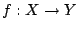, one has an induced map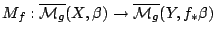from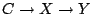. If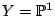, then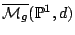is a compactification of the space of branched covers. We want to show that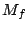is surjective. (We only need the coarse moduli space; in fact, at least in characteristic zero we have a Deligne-Mumford stack.)

Whenis, the boundis sharp. Ifis not algebraically closed, then pick a finite extension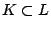, and consider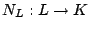; this has a polynomial of degree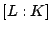with no nontrivial zeros; therefore it is impossible to get a larger class of hypersurfaces.

Is the notion of rational connectivity sharp? In the case of finite fields, we also get: ifsmooth projective, and either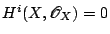for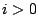or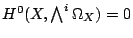for, thenhas a rational point. (These two are equivalent in characteristic zero.) The analog over function fields is false. In particular, there exists a familyof Enriques surfaces over(is a curve over) which has no section.

The general statement: Let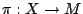be a proper morphism of varieties over. Suppose that for all maps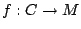, there exists a pullback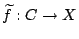. It would suffice for there to exist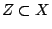dominating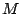such that the general fibre of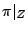is rationally connected. It is a theorem that this is necessary and sufficient (G, Harris, Mazur, Starr).

Back to the main index for Rational and integral points on higher dimensional varieties.